• Library:
• DSP System Toolbox / Filtering / Filter Implementations

DSP System Toolbox HDL Support / Filtering

•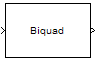## Description

The Biquad Filter block independently filters each channel of the input signal with the specified biquadratic infinite impulse response (IIR) filter. When you specify the filter coefficients in the dialog box, the block implements static filters with fixed coefficients. When you provide the filter coefficients through an input port, you can tune the coefficients during simulation.

## Ports

### Input

expand all

Data input to the block, specified as a vector or a matrix. This block supports variable-size input signals, enabling you to change the input frame size (number of rows) during simulation. However, the number of channels (number of columns) must remain constant.

If the input is fixed-point, it must be signed fixed-point with binary point scaling.

This port is unnamed unless you set the Coefficient source to `Input port(s)`.

Data Types: `single` | `double` | `int8` | `int16` | `int32` | `int64` | `fixed point`
Complex Number Support: Yes

Numerator coefficients of the biquad filter, specified as a 3-by-N matrix, where N is the number of biquad filter sections.

If is fixed-point, it must be signed fixed-point with binary point scaling.

#### Dependencies

This port appears only when you set the Coefficient source to `Input port(s)`.

Data Types: `single` | `double` | `int8` | `int16` | `int32` | `int64` | `fixed point`

Denominator coefficients of the biquad filter, specified as a 2-by-N matrix, where N is the number of biquad filter sections.

If is fixed-point, it must be signed fixed-point with binary point scaling.

#### Dependencies

This port appears only when you set the Coefficient source to `Input port(s)`.

Data Types: `single` | `double` | `int8` | `int16` | `int32` | `int64` | `fixed point`

Scale values of the biquad filter, specified as a 1-by-(N+1) vector, where N is the number of biquad filter sections.

If is fixed-point, it must be signed fixed-point with binary point scaling.

#### Dependencies

This port appears only when you set the Coefficient source to `Input port(s)`.

Data Types: `single` | `double` | `int8` | `int16` | `int32` | `int64` | `fixed point`

### Output

expand all

Filtered output, returned as a vector or a matrix.

The output dimensions always equal the dimensions of the input signal. The output of this block numerically matches the outputs of the `dsp.BiquadFilter` System object™.

If is fixed-point, it must be signed fixed-point with binary point scaling.

This port is unnamed unless you set the Coefficient source to `Input port(s)`.

Data Types: `single` | `double` | `int8` | `int16` | `int32` | `int64` | `fixed point`
Complex Number Support: Yes

## Parameters

expand all

### Main Tab

The Biquad Filter block can operate in three different modes:

• `Dialog parameters` — Enter information about the filter, such as structure and coefficients, in the block mask.

• `Input port(s)` — Enter information about the filter structure in the block mask using the Filter structure parameter. The filter coefficients come into the block through additional input ports that appear on the block icon:

• `Num` — Specify numerator coefficients.

• `Den` — Specify denominator coefficients.

• `g` — Specify scale values.

The block assumes the first denominator coefficients and of each section to be 1. This configuration is applicable when the `SOSMatrixSource` property is `'Input port'` and the `ScaleValuesInputPort` property is `true`. The reason you would need to specify Num and Den instead of the SOSMatrix, is that in Fixed-Point operation, the numerators, and denominators can have different fraction lengths. Therefore, there is a need to be able to pass the data of the numerator with a fixed-point type different from that of the denominator.

• `Filter object` — Specify the filter using a `dsp.BiquadFilter` System object.

Specify the name of the discrete-time filter that you want the block to implement. You must specify the filter as a `dsp.BiquadFilter` System object.

You can define the System object in the block mask or in a MATLAB® workspace variable.

For information on creating System objects, see Define Basic System Objects.

#### Dependencies

This parameter is visible only when Coefficient source is set to `Filter object`.

Specify the filter structure.

#### Dependencies

This parameter is visible only when Coefficient source is set to `Dialog parameters` or ```Input port(s)```.

Specify an M-by-6 matrix, where M is the number of sections in the second-order section filter. Each row of the SOS matrix contains the numerator and denominator coefficients (bik and aik) of the corresponding section in the filter.

`$\left[\begin{array}{cccccc}{b}_{01}& {b}_{11}& {b}_{21}& {a}_{01}& {a}_{11}& {a}_{21}\\ {b}_{02}& {b}_{12}& {b}_{22}& {a}_{02}& {a}_{12}& {a}_{22}\\ ⋮& ⋮& ⋮& ⋮& ⋮& ⋮\\ {b}_{0M}& {b}_{1M}& {b}_{2M}& {a}_{0M}& {a}_{1M}& {a}_{2M}\end{array}\right]$`

The leading denominator coefficients [a01 a02 ... a0N] are treated as 1s, regardless of their actual values. No scaling is applied to the SOS matrix when a0 is not 1.

The `ss2sos` and `tf2sos` functions convert a state-space or transfer function description of your filter into the second-order section description used by this block.

#### Dependencies

This parameter is visible only when Coefficient source is set to `Dialog parameters`.

Specify scale values to be used between SOS sections. You can specify a real-valued scalar or a vector of length M+1:

• When you enter a scalar, the value specifies the gain value before the first section of the second-order filter. The rest of the gain values default to 1.

• When you enter a vector of M+1 values, each value specifies a separate section of the filter. For example, the first element is the first gain value, the second element is the second gain value, and so on.Select the Optimize unity scale values check box to optimize your simulation when one or more scale values equal 1. Selecting this option removes the unity gains so that the values are treated like Simulink lines or wires. In some fixed-point cases when there are unity scale values, selecting this parameter also omits certain casts. Refer to the Fixed-Point Conversion section under Extended Capabilities for more information.

#### Dependencies

This parameter is visible only when Coefficient source is set to `Dialog parameters`.

Specify the initial conditions of the filter states when Filter structure is set to `Direct form II` or `Direct form II transposed`.

Direct form II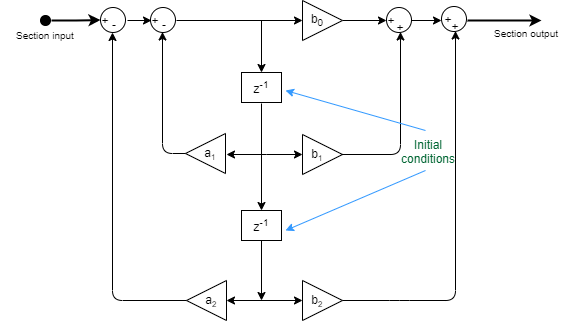Direct form II transposed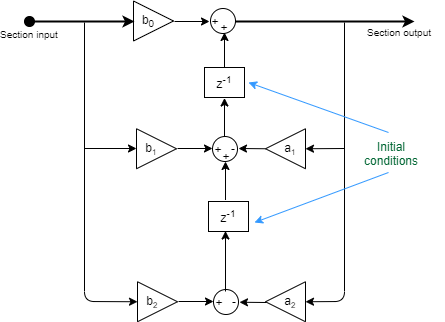The Biquad Filter block initializes the internal filter states to zero by default. To specify nonzero initial states for the filter delays, use the Initial conditions parameter.

To determine the number of initial conditions you must specify and how to specify them, see the following table on valid initial conditions.

Valid Initial Conditions

Initial ConditionDescription

Scalar

The block initializes all delay elements in the filter to the scalar value.

Vector or matrix
(for applying different delay elements to each channel)

Each vector or matrix element specifies a unique initial condition for a corresponding delay element in a corresponding channel. M is the number of sections, and N is the number of input channels:

• The vector length must equal the number of delay elements in the filter, 2M.

• The matrix must have the same number of rows as the number of delay elements in the filter 2MN. The matrix must also have one column for each channel of the input signal.

#### Dependencies

This parameter is only visible when Coefficient source is set to `Dialog parameters` or `Input port(s)` and the Filter structure is set to ```Direct form II``` or `Direct form II transposed`.

Specify the initial conditions for the filter states on the side of the filter structure with the zeros (b0, b1, b2, …). This parameter applies only when Filter structure is set to `Direct form I` or ```Direct form I transposed```.

Direct form I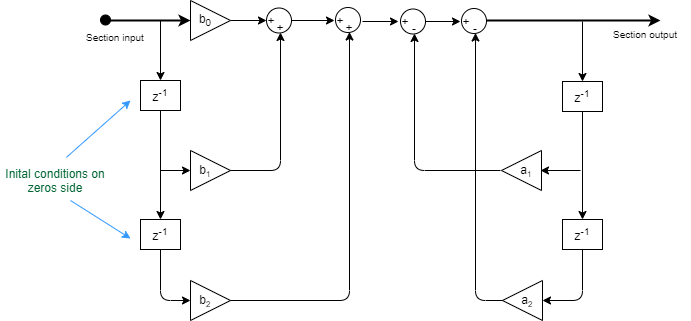Direct form I transposed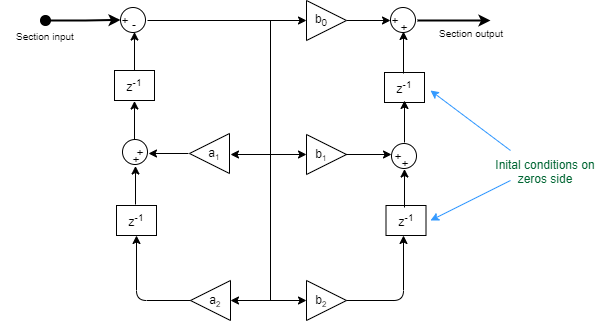The Biquad Filter block initializes the internal filter states to zero by default. To specify nonzero initial states for the filter delays, use the Initial conditions on zeros side parameter.

For an example model, type the following model name in the MATLAB command prompt.

`ex_biquad_filter_ref`

To determine the number of initial conditions you must specify and how to specify them, see the following table on valid initial conditions.

Valid Initial Conditions

Initial ConditionDescription

Scalar

The block initializes all delay elements in the filter to the scalar value.

Vector or matrix
(for applying different delay elements to each channel)

Each vector or matrix element specifies a unique initial condition for a corresponding delay element in a corresponding channel. Where M is the number of sections and N is the number of input channels:

• The vector length must equal the number of delay elements in the filter, 2M.

• The matrix must have the same number of rows as the number of delay elements in the filter 2MN. The matrix must also have one column for each channel of the input signal.

#### Dependencies

This parameter is visible only when Coefficient source is set to `Dialog parameters` or `Input port(s)` and the Filter structure is set to ```Direct form I``` or `Direct form I transposed`.

Specify the initial conditions for the filter states on the side of the filter structure with the poles (a1, a2, ...). This parameter applies only when Filter structure is set to `Direct form I` or `Direct form I transposed`.

Direct form I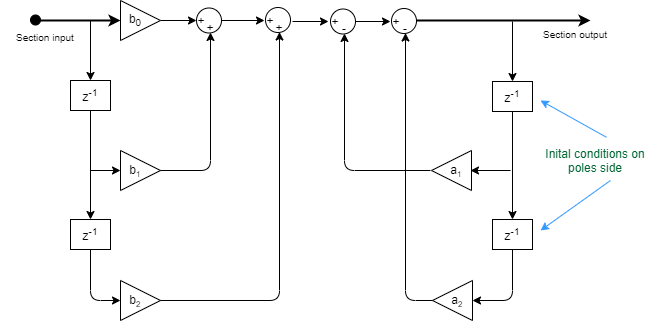Direct form I transposed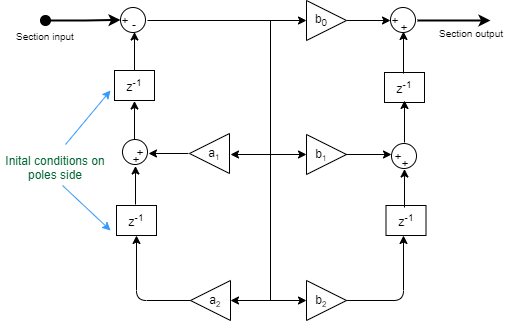The Biquad Filter block initializes the internal filter states to zero by default. To specify nonzero initial states for the filter delays, use the Initial conditions on poles side parameter.

For an example model, type the following model name in the MATLAB command prompt.

`ex_biquad_filter_ref`

To determine the number of initial conditions you must specify and how to specify them, see the following table on valid initial conditions.

Valid Initial Conditions

Initial ConditionDescription

Scalar

The block initializes all delay elements in the filter to the scalar value.

Vector or matrix
(for applying different delay elements to each channel)

Each vector or matrix element specifies a unique initial condition for a corresponding delay element in a corresponding channel. Where M is the number of sections and N is the number of input channels:

• The vector length must equal the number of delay elements in the filter, 2M.

• The matrix must have the same number of rows as the number of delay elements in the filter 2MN. The matrix must also have one column for each channel of the input signal.

#### Dependencies

This parameter is visible only when Coefficient source is set to `Dialog parameters` or `Input port(s)` and the Filter structure is set to ```Direct form I``` or `Direct form I transposed`.

Choose how to specify the scale values to use between filter sections. When you select `Specify via input port (g)`, you enter the scale values as a 2-D vector at port g. When you select ```Assume all are unity and optimize```, all scale values are removed and treated like Simulink lines or wires.

#### Dependencies

This parameter is visible only when Coefficient source is set to `Input port(s)`.

Specify the action the block should perform when the SOS matrix a0j values do not equal one. The action can be `Warning`, `Error`, or `None`.

When you choose `None`, the leading coefficients a0j are treated as 1's, regardless of their actual values. No scaling is applied on the SOS matrix when a0 is not 1.

#### Dependencies

This parameter is visible only when Coefficient source is set to `Dialog parameters`.

Select this check box to optimize your simulation when one or more scale values equal 1. Selecting this option removes the unity gains so that the values are treated like Simulink lines or wires. In some fixed-point cases when there are unity scale values, selecting this parameter also omits certain casts. See the Fixed Point section under Extended Capabilities for more information.

#### Dependencies

This parameter is visible only when Coefficient source is set to `Dialog parameters`.

Specify how the block should process the input. If the input is an M-by-N matrix, you can set this parameter to:

• `Columns as channels (frame based)` (default) — The block treats each column as a separate channel. In this mode, the block creates M instances of the same filter, each with its own independent state buffer. Each of the M filters process N input samples at every Simulink time step.

• `Elements as channels (sample based)` — The block treats each element as a separate channel. In this mode, the block creates MN instances of the same filter, each with its own independent state buffer. Each filter processes one input sample at every Simulink time step.

This button opens the Filter Visualization Tool (`fvtool`) and displays the filter response of the filter specified in the dialog.

Note

When you make changes to the filter parameters on the block dialog, you must click the button before using the button.

### Data Types Tab

Note

This tab appears only when you set Coefficient source to either `Dialog parameters` or `Input port(s)`. When the Coefficient source is set to `Filter object`, the data types specified in the filter object properties are used by the block.

Specify the rounding mode for fixed-point operations.

For more details, see rounding mode. The filter coefficients do not obey this parameter; instead, they always round to `Nearest`.

When you select this parameter, the block saturates the result of its fixed-point operation. When you clear this parameter, the block wraps the result of its fixed-point operation. For details on `saturate` and `wrap`, see overflow mode for fixed-point operations.

The filter coefficients are always saturated and do not obey this parameter.

Choose how you specify the word and fraction lengths of the fixed-point data type going into each section of a biquadratic filter. See the Fixed-Point Conversion section under Extended Capabilities for illustrations depicting the use of the section input data type in this block. When you select:

• `Same as input` — Word length and fraction length characteristics of the Section input data type match those of the input to the block.

• `Binary point scaling` — Enter the word and fraction lengths of the section input, in bits.

Choose how you specify the word and fraction lengths of the fixed-point data type coming out of each section of a biquadratic filter. See the Fixed-Point Conversion section under Extended Capabilities for illustrations depicting the use of the section output data type in this block. When you select:

• `Same as section input` — Word length and fraction length characteristics of the Section output data type match with those of the input to the block.

• `Binary point scaling` — Enter the word and fraction lengths of the section output, in bits.

Choose how you specify the word and fraction lengths of the multiplicand data type of a `Direct form I transposed` filter structure. See the Fixed-Point Conversion section under Extended Capabilities for illustrations depicting the use of the multiplicand data type in this block.

When you select:

• `Same as output` — Word length and fraction length characteristics of the Multiplicand data type match with those of the output of the block.

• `Binary point scaling` — Enter the word length and the fraction length of the multiplicand, in bits.

#### Dependencies

This parameter is visible only when the Filter structure parameter is set to `Direct form I transposed`.

Choose how you specify the word and fraction lengths of the filter coefficients (numerator, denominator, and scale value) when Coefficient source is set to `Dialog parameters`. See the Fixed-Point Conversion section under Extended Capabilities for illustrations depicting the use of the coefficient data types in this block. When you select:

• `Same word length as input` — Word length of the filter coefficients matches that of the input to the block. In this mode, the block automatically sets the fraction length of the coefficients to the binary point-only scaling that provides the best precision possible given the value and word length of the coefficients.

• `Specify word length` — Enter the word length of the coefficients, in bits. In this mode, the block automatically sets the fraction length of the coefficients to the binary point-only scaling that provides the best precision possible given the value and word length of the coefficients.

• `Binary point scaling` — Enter the word length and the fraction length of the coefficients, in bits. If applicable, enter separate fraction lengths for the numerator and denominator coefficients.

The filter coefficients do not obey the Rounding mode and the Overflow mode parameters; instead, they are always saturated and rounded to `Nearest`.

#### Dependencies

This parameter is visible only when Coefficient source is set to `Dialog parameters`.

Specify how to designate the product output word and fraction lengths. See Multiplication Data Types and the Fixed-Point Conversion section under Extended Capabilities for illustrations depicting the use of the product output data type in this block. When you select:

• `Same as input` — Product output word length and fraction length characteristics match those of the input to the block.

• `Inherit via internal rule` — Product output word length and fraction lengths are computed based on full-precision rules. These rules prevent quantization from occurring within the block. Bits are added, as needed, so that no roundoff or overflow occurs. For more details, see Inherit via Internal Rule.

• `Binary point scaling` — Enter the word length and the fraction length of the product output, in bits. If applicable, enter separate fraction lengths for the numerator and denominator product output data type.

Specify how to designate the accumulator word and fraction lengths. See Multiplication Data Types and the Fixed-Point Conversion section under Extended Capabilities for illustrations depicting the use of the accumulator data type in this block. When you select:

• `Same as input` — Accumulator word and fraction length characteristics match those of the input to the block.

• `Same as product output` — Accumulator word and fraction length characteristics match those of the product output.

• `Binary point scaling` — Enter the word length and the fraction length of the accumulator, in bits. If applicable, enter separate fraction lengths for the numerator and denominator accumulator data type.

Specify how to designate the state word and fraction lengths when Coefficient source is set to `Dialog parameters`. See the Fixed-Point Conversion section under Extended Capabilities for illustrations depicting the use of the state data type in this block.

When you select:

• `Same as input` — State word and fraction length characteristics match those of the input to the block.

• `Same as accumulator` — State word and fraction length characteristics match those of the accumulator.

• `Binary point scaling` — Enter the word length and the fraction length of the state, in bits. If applicable, enter separate fraction lengths for the numerator and denominator state data type.

#### Dependencies

This parameter is visible only when Filter structure is set to `Direct form II` or ```Direct form II transposed```.

Choose how you specify the output word length and fraction length. See the Fixed-Point Conversion section under Extended Capabilities for illustrations depicting the use of the output data type in this block. When you select:

• `Same as input` — Output word and fraction length characteristics match those of the input to the block.

• `Same as accumulator` — Output word and fraction length characteristics match those of the accumulator.

• `Binary point scaling` — Enter the word length and the fraction length of the output, in bits.

Select this parameter to prevent the fixed-point tools from overriding the data types you specify on the block mask.

## Block Characteristics

 Data Types `double` | `fixed point` | `integer` | `single` Direct Feedthrough `no` Multidimensional Signals `no` Variable-Size Signals `yes` Zero-Crossing Detection `no`

## Extended Capabilities

### Blocks

Introduced in R2008b

## SupportGet trial now